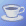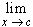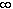#Interactive Real Analysis

## 6. Limits, Continuity, and Differentiation

### 6.2. Continuous Functions

If one looks up continuity in a thesaurus, one finds synonyms like perpetuity or lack of interruption. Descartes said that a function is continuous if its graph can be drawn without lifting the pencil from the paper.
 Example 6.2.1:Use the above imprecise meaning of continuity to decide which of the two functions are continuous: f(x) = 1 if x > 0 and f(x) = -1 if x < 0. Is this function continuous ? f(x) = 5x - 6. Is this function continuous?
However, if we want to deal with more complicated functions, we need mathematical concepts that we can manipulate.
 Definition 6.2.2: Continuity A function is continuous at a point c in its domain D if: given any> 0 there exists a> 0 such that: if xD and | x - c | <then | f(x) - f(c) | <A function is continuous in its domain D if it is continuous at every point of its domain.This, like many epsilon-delta definitions and arguments, is not easy to understand. Click on the Java icon to see an applet that tries to illustrate the definition.

Continuous functions are precisely those groups of functions that preserve limits, as the next proposition indicates:

 Proposition 6.2.3: Continuity preserves Limits If f is continuous at a point c in the domain D, and { xn } is a sequence of points in D converging to c, thenf(x) = f(c). Iff(x) = f(c) for every sequence { xn } of points in D converging to c, then f is continuous at the point c.
Again, as with limits, this proposition gives us two equivalent mathematical conditions for a function to be continuous, and either one can be used in a particular situation.
 Example 6.2.4:Which of the following two functions is continuous: If f(x) = 5x - 6, prove that f is continuous in its domain. If f(x) = 1 if x is rational and f(x) = 0 if x is irrational, prove that x is not continuous at any point of its domain.If f(x) = x if x is rational and f(x) = 0 if x is irrational, prove that f is continuous at 0.If f(x) is continuous in a domain D, and { xn } is a Cauchy sequence in D, is the sequence { f ( xn ) } also Cauchy ?
Continuous functions can be added, multiplied, divided, and composed with one another and yield again continuous functions.
 Proposition 6.2.5: Algebra with Continuous Functions The identity function f(x) = x is continuous in its domain. If f(x) and g(x) are both continuous at x = c, so is f(x) + g(x) at x = c. If f(x) and g(x) are both continuous at x = c, so is f(x) * g(x) at x = c. If f(x) and g(x) are both continuous at x = c, and g(x) # 0, then f(x) / g(x) is continuous at x = c. If f(x) is continuous at x = c, and g(x) is continuous at x = f(c), then the composition g(f(x)) is continuous at x = c.
While this proposition seems not very important, it can be used to quickly prove the following:
 Examples 6.2.6:Every polynomial is continuous in R, and every rational function r(x) = p(x) / q(x) is continuous whenever q(x) # 0.The absolute value of any continuous function is continuous.
Continuity is defined at a single point, and the epsilon and delta appearing in the definition may be different from one point of continuity to another one. There is, however, another kind of continuity that works for all points of domain at the same time.
 Definition 6.2.7: Uniform Continuity A function f with domain D is called uniformly continuous on the domain D if for any> 0 there exists a> 0 such that: if s, tD and | s - t | <then | f(s) - f(t) | <. Click here for a graphical explanation.Take a look at this Java applet illustrating uniform continuity.

While this definition looks very similar to the original definition of continuity, it is in fact not the same: a function can be continuous, but not uniformly continuous. The difference is that the delta in the definition of uniform continuity depends only on epsilon, whereas in the definition of simply continuity delta depends on epsilon as well as on the particular point c in question.

 Example 6.2.8:The function f(x) = 1 / x is continuous on (0, 1). Is it uniformly continuous there ?The function f(x) = x2 is continuous on [0, 1]. Is it uniformly continuous there ?The function f(x) = x2 is continuous on [0,). Is it uniformly continuous there ?If f(x) is uniformly continuous in R, and { xn } is a Cauchy sequence, is the sequence { f ( xn ) } also Cauchy ?
The next theorem illustrates the connection between continuity and uniform continuity, and gives an easy condition for a continuous function to be uniformly continuous.
 Theorem 6.2.9: Continuity and Uniform Continuity If f is uniformly continuous in a domain D, then f is continuous in D. If f is continuous on a compact domain D, then f is uniformly continuous in D.

Next, we will look at functions that are not continuous.